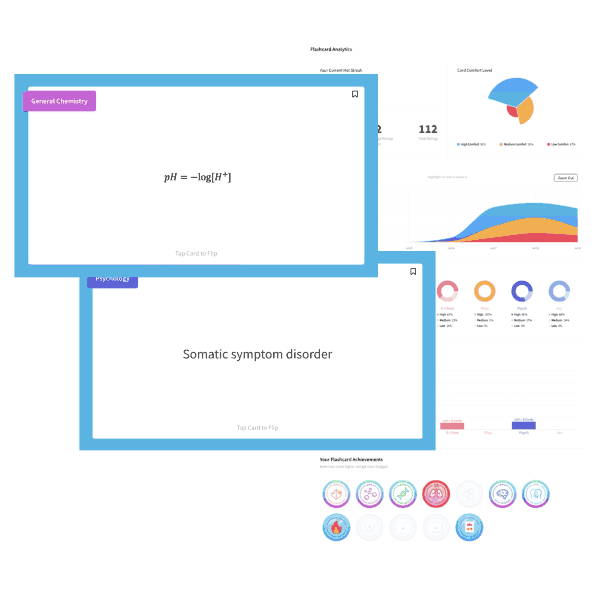# MCAT Chem Practice Question – Ion to Atoms Ratio

A 1-L beaker is filled with 2 moles of liquid methanol and 0.25 moles of NaCl. The ratio of ions to atoms in this solution is:

A. 0.5:2

B. 1:6

C. 1:25

D. 3:1

Explanation

C is correct. Atoms are the tiny units that compose matter; they consist of electron(s) and a central nucleus. The term “ion” refers specifically to charged atoms. Luckily, the number of moles of a substance directly relates to the number of particles by way of Avogadro’s number, so a direct comparison of moles is all we need to find our answer. However, two moles of a molecule is not equivalent to two moles of atoms. For example, since methanol has a formula of CH3OH, it contains a total of six atoms per molecule. In contrast, NaCl will dissociate into two ions: Na+ and Cl. In this solution, we have 0.25 moles of NaCl, which can be thought of as (0.25 moles * 2) or 0.5 ions. For atoms, we have (2 moles * 6) + (0.25 * 2) or 12.5; remember that ions are technically atoms as well! This gives us a ratio of 0.5:12.5 or 1:25.

A. 0.5:2: incorrect. This is simply the ratio of moles of ions to moles of methanol.

B. 1:6; incorrect. This is the ratio of molecules of NaCl to atoms in methanol, but neglects to involve moles or account for the two ions that NaCl produces.

D. 3:1; incorrect. This answer is incorrect in several ways. It likely was derived from a 6:2 ratio, or the ratio of atoms in methanol to ions in NaCl. However, this question asks for the ion-to-atom ratio. Additionally, this answer does not account for the moles present.

## Want more MCAT practice?

### We’ve got options for every schedule and learning style!

From the best online MCAT course created by top instructors with 524+ MCAT scores to the most representative full-length practice exams and private tutoring, we can custom tailor your MCAT prep to your goals!

Not sure which option is right for you? Schedule a free MCAT consultation with an MCAT expert using the form below. No obligation, just expert advice.

Schedule My Free Consultation